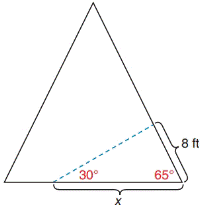Chapter 11.4, Problem 33EElementary Geometry For College St...

7th Edition
Alexander + 2 others
ISBN: 9781337614085

Solutions

Chapter
SectionElementary Geometry For College St...

7th Edition
Alexander + 2 others
ISBN: 9781337614085
Textbook Problem

In Exercises 29 to 34, use the Law of Sines or the Law of Cosines to solve each problem. Angle measures should be found to the nearest degree and areas and distances to the nearest tenth of a unit.In an A-frame house, a bullet is found embedded at a point 8  ft up the sloped wall. If it was fired at a 30 ∘ angle with the horizontal, how far from the base of the wall was the gun fired?To determine

To find:

The distance from the base of the wall was the gun fired.

Explanation

1) Approach:

The Law of Sines,

sinαa=sinβb=sinγc

Where α, β, and γ are the acute angles of the triangle, and a, b, and c are the sides of the triangle.

2) Calculation:

Given,

In an A-frame house, a bullet is found embedded at a point 8 ft up the sloped wall if it was fired at a 30 angle with the horizontal.

From the figure:

Here, AC¯=b=8ft, BC=a=x,

B=30and C = 65

(i.e.) b=8ft, a=x, β=30 and γ=65

Find x:

For any triangle

α+β+γ=180

α+30+65=180

α+95=180

Add 95 to both sides

Still sussing out bartleby?

Check out a sample textbook solution.

See a sample solution

The Solution to Your Study Problems

Bartleby provides explanations to thousands of textbook problems written by our experts, many with advanced degrees!

Get Started

Factoring Factor the expression completely. 34. 7x3/2 8x1/2 + x1/2

Precalculus: Mathematics for Calculus (Standalone Book)

Calculate y'. 17. y=arctan

Single Variable Calculus: Early Transcendentals, Volume I

Solve the equations in Exercises 126. x44x2=4

Finite Mathematics and Applied Calculus (MindTap Course List)

For the area of the shaded region, which integral form, dx or dy, should be used? a) dx b) dy c) Either...

Study Guide for Stewart's Single Variable Calculus: Early Transcendentals, 8th

, where E is the wedge-shaped solid shown at the right, equals:

Study Guide for Stewart's Multivariable Calculus, 8th